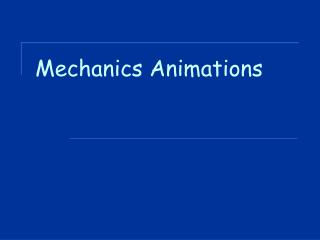DownloadDownload PresentationMechanics Animations

# Mechanics Animations

Télécharger la présentation## Mechanics Animations

- - - - - - - - - - - - - - - - - - - - - - - - - - - E N D - - - - - - - - - - - - - - - - - - - - - - - - - - -
##### Presentation Transcript

1. Mechanics Animations

2. Average v Instantaneous Speed Instantaneous speed of your car is your speed at a particular instant in time. Average speed is a measure of the distance travelled in a given period of time

3. Velocity & acceleration vectors The velocity and acceleration of the car are depicted by vector arrows.

4. Acceleration • Which car or cars (red, green, and/or blue) are undergoing an acceleration? • Which car (red, green, or blue) experiences the greatest acceleration? • Match the appropriate line on the graph to the particular colour of car. • ANSWERS • The green and blue cars • The blue car • The red car is line B, the green car is line C, the blue car is line A

5. Position v time, velocity v time, acceleration v time graphs Constant Positive Velocity

6. Constant negative velocity

7. Positive Velocity and Positive Acceleration

8. Positive Velocity and Negative Acceleration

9. Negative Velocity and Negative Acceleration

10. Negative Velocity and Positive Acceleration

11. Overtaking cars: Position v time • The position-time plot of each car's motion is depicted by a diagonal line with a constant slope. • This diagonal line is shows constant velocity. • When the cars are side by side, the lines intersect. The two cars have the same position at seven seconds. • The cars never have the same velocity at any instant in time.

12. Overtaking cars: Velocity v time • Each car's motion is represented by a horizontal line, indicating a constant velocity. • Even though the cars pass each other, the lines on the velocity-time graphs do not intersect. • Since the cars never have the same velocity, the lines on the velocity-time graph never cross.

13. What is the final velocity of a car that accelerates from rest at 4 m/s2 for 3 seconds? • What is the displacement of each car after 3 seconds? (Use the area of the velocity-time graph.) • What is the gradient of the line for the red car for the first 3 seconds? • What is the displacement of each car after 9 seconds? • Does the red car pass the blue car at 3 seconds? If not, then when does the red car pass the blue car? • When lines on a velocity-time graph intersect, does it mean that the two cars are passing by each other? If not, what does it mean?

14. Answers • 12 m/s • Red Car: Area of Triangle = 0.5*b*h = 0.5*(3 s)*(12 m/s)=18 m • Blue Car: Area of Rectangle = b*h = (3 s)*(10 m/s) = 30 m • Gradient = rise/run = (12 m/s- 0 m/s) / (3 s) = 4 m/s/s • Red Car: Area of Triangle + Area of Rectangle = 0.5*b1*h1 + b2*h2 = 0.5*(3 s)*(12 m/s) +(9 s)*(12 m/s) = 18 m + 72 m = 90 m • Blue Car: Area of Rectangle = b*h = (9 s)*(10 m/s) = 90 m • No! The red car passes the blue car at 9 seconds. See animation and the result of the above question. • No! When lines intersect on a velocity-time graph, it means that the two cars have the same velocity. When lines intersect on a position-time graph, it means that the two cars are passing each other.

15. Freefall: True or False • Both objects fall at the same rate as they have the same mass • The elephant has a greater acceleration (due to gravity) than the feather. • The elephant has more mass, yet both elephant and feather experience the same weight. • On earth, all objects (whether an elephant or a feather) have the same weight. All statements are FALSE

16. Freefall: with air resistance

17. Freefall

18. Energy conversion: roller coaster KE = total kinetic energy PE= total gravitational potential energy TME= total mechanical energy

19. Energy conversion: pendulum